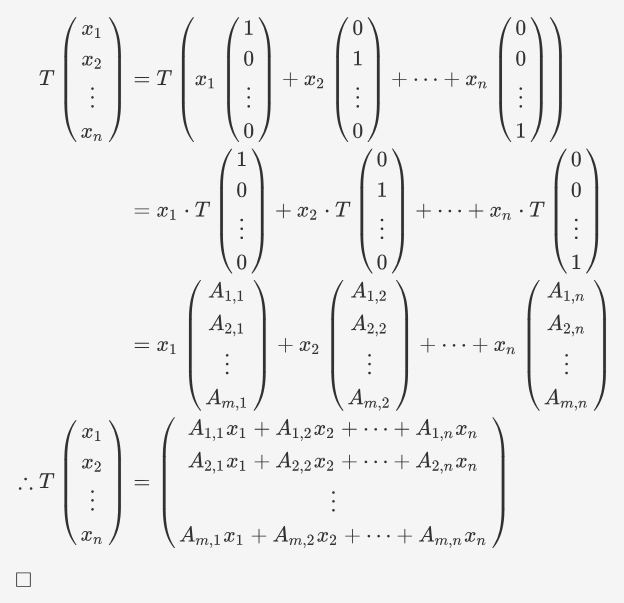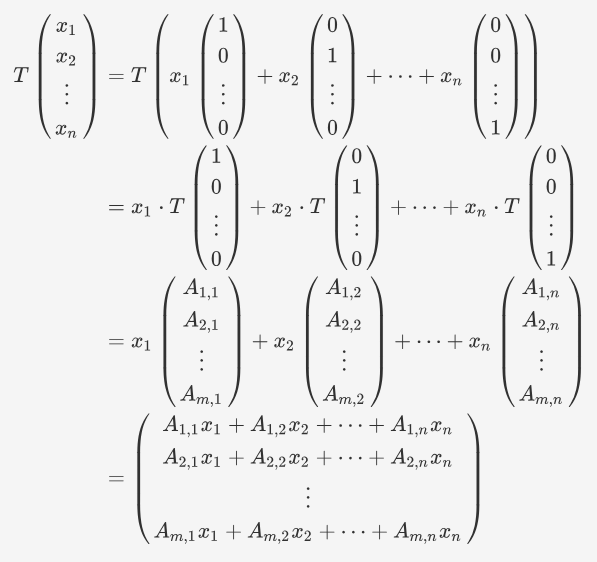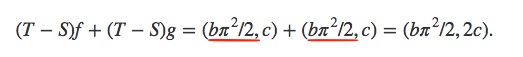If you find any mistakes, please make a comment! Thank you.

## Chapter 3 Exercise A

1. Solution: If $T$ is linear, then $(0,0)=T(0,0,0)=(b,0)$by 3.11, hence $b=0$. We also have $T(1,1,1)=T(1,1,0)+T(0,0,1),$it is equivalent to $(1+b,6+c)=(b-2,6)+(3+b,0)=(1+2b,6).$Thus $6+c=6$ implies $c=0$.

Conversely, if $b=c=0$, $T$ is obviously linear. See 3.4 or Problem 3.

You may consider a linear map only has linear terms, hence the terms like $xyz$ is impossible.

2. Solution: We show that if $b=c=0$, then $T$ is linear. Let $f,g\in \ca P(\R)$, then we have $(f+g)(4)=f(4)+g(4)$and $(f+g)'(4)=f'(4)+g'(4).$It is easy to check. Moreover, by linearity of integration, one has $\int_{-1}^2x^3(f+g)(x)dx=\int_{-1}^2x^3(f(x)+g(x))dx=\int_{-1}^2x^3f(x)dx+\int_{-1}^2x^3g(x)dx.$ By the above, it follows that \begin{align*} T(f+g)=&(3(f+g)(4)+5(f+g)'(6),\int_{-1}^2x^3(f+g)(x)dx)\\ =&(3f(4)+5f'(6),\int_{-1}^2x^3f(x)dx)+(3g(4)+5g'(6),\int_{-1}^2x^3g(x)dx)\\ =&Tf+Tg. \end{align*} Similarly, we can check that $T(\lambda f)=\lambda Tf$ for any $\lambda\in \R$ and $f\in \ca P(\R)$. Conversely, denote the linear map above by $S$. Then by Problem 5, it follows that $T-S$ is a linear map. That means $(T-S)p=(bp(1)p(2),c\sin p(0))$is linear. Consider $f(x)=\pi/2$ and $g(x)=\pi/2$, then $f,g\in\ca P(\R)$. We have $(T-S)(f+g)=(b\pi^2,c\sin \pi)=(b\pi^2,0)$and $(T-S)f+(T-S)g=(b\pi^2/4,c)+(b\pi^2/4,c)=(b\pi^2/2,2c).$Thus, we should have $(b\pi^2,0)=(b\pi^2/2,2c).$It follows that $b=c=0$.

We do not really need Problem 5, we need them just for simplifying computation.

3. Solution: If we denote $T(1,0,\cdots,0)=(A_{1,1},\cdots,A_{m,1}),$$T(0,1,\cdots,0)=(A_{1,2},\cdots,A_{m,2}),$$\cdots\cdots$and$T(0,0,\cdots,0,1)=(A_{1,n},\cdots,A_{m,n}).$Note that $(1,0,\cdots,0)$, $(0,1,\cdots,0)$, $\cdots$ and $(0,\cdots,0,1)$ is a basis of $\mb F^n$, then by the proof of 3.5 we conclude that $T(x_1,\cdots,x_n)=(A_{1,1}x_1+\cdots+A_{1,n}x_n,\cdots,A_{m,1}x_1+\cdots+A_{m,n}x_n).$

4. Solution: Suppose there are $a_1$, $\cdots$, $a_m\in\mb F$ such that $0=a_1v_1+\cdots+a_mv_m,$then $0=T(a_1v_1+\cdots+a_mv_m)=a_1Tv_1+\cdots+a_mTv_m.$Note that $T v_1$, $\cdots$, $T v_m$ is linearly independent, it follows that $a_1=\cdots=a_m=0,$thus $v_1$, $\cdots$, $v_m$ is linearly independent.

5. Solution: It suffices to show that $T+S$ and $\lambda T$ are linear maps provided $T$ and $S$ are linear maps, where $\lambda \in\mb F$. Then $\ca L(V,W)$ is closed under addition and scalar multiplication, hence is a vector space.

For any $u,v\in V$, we have \begin{align*} (T+S)(u+v)=&T(u+v)+S(u+v)=Tu+Tv+Su+Sv\\ =&(Tu+Su)+(Tv+Sv)=(T+S)u+(T+S)v. \end{align*} The first and last equality hold by the definitions in 3.6, the second equality holds since $T$ and $S$ are linear maps. Similarly, for $\eta\in\mb F$, \begin{align*} (T+S)(\eta u)=&T(\eta u)+S(\eta u)=\eta Tu+\eta Su\\ =&\eta(Tu+Su)=\eta(T+S)u. \end{align*} Combining these arguments, it follows that $T+S$ is a linear map. Again, for any $u,v\in V$, we have \begin{align*} (\lambda T)(u+v)=&\lambda (T(u+v))=\lambda (Tu+Tv)\\ =&\lambda (Tu)+\lambda (Tv)=(\lambda T)u+(\lambda T)v. \end{align*} The first and last equality hold by the definitions in 3.6, the second equality holds since $T$ is a linear map. Similarly, for $\eta\in\mb F$, \begin{align*} (\lambda T)(\eta u)=&\lambda(T(\eta u))=\lambda(\eta T( u))\\ =&\lambda\eta(Tu)=\eta(\lambda Tu)=\eta(\lambda T)u. \end{align*} Combining these arguments, it follows that $\lambda T$ is a linear map.

6. Solution: Associativity: by definition, for any $x\in V$, we have $((T_1T_2)T_3)x=(T_1T_2)(T_3x)=T_1(T_2(T_3x)),$while $(T_1(T_2T_3))x=T_1((T_2T_3)x)=T_1(T_2(T_3x)).$Hence $((T_1T_2)T_3)x=(T_1(T_2T_3))x$ for any $x\in V$, therefore $(T_1T_2)T_3=T_1(T_2T_3)$.

Identity: for any $x\in V$, we have $(TI)x=T(Ix)=Tx$while$(IT)x=I(Tx)=Tx.$Hence $(IT)x=(TI)x=Tx$ for any $x\in V$, therefore $IT=TI=T$.

Distributive properties: we only show $(S_1+S_2)T=S_1T+S_2T$. For any $u\in U$, we have $((S_1+S_2)T)u=(S_1+S_2)(Tu)=S_1(Tu)+S_2(Tu),$while $(S_1T+S_2T)u=(S_1T)u+(S_2T)u=S_1(Tu)+S_2(Tu).$ Hence $((S_1+S_2)T)u=(S_1T+S_2T)u$ for any $u\in U$, therefore $(S_1+S_2)T=S_1T+S_2T$. Similarly, we can show $S(T_1+T_2)=ST_1+ST_2$.

7. Solution: See Linear Algebra Done Right Solution Manual Chapter 3 Problem 1.

8. Solution: See Linear Algebra Done Right Solution Manual Chapter 3 Problem 2.

9. Solution: Consider the function defined by $\vp(a+bi)=a$, where $a,b\in\R$. If $w=\alpha_1+\beta_1 i$ and $z=\alpha_2+\beta_2 i$, where $\alpha_1$, $\alpha_2$, $\beta_1$ and $\beta_2$ are real numbers, then we have $\vp(w+z)=\vp(\alpha_1+\beta_1 i+\alpha_2+\beta_2 i)=\alpha_1+\alpha_2=\vp(w)+\vp(z).$However, $i\vp(1)=i\ne \vp(i\cdot 1)=0,$hence $\vp$ is not linear over $\C$.

For $\vp:\R\to\R$ case, please see On sort-of-linear functions.

10. Solution: Note that $U\ne V$ and $S\ne 0$, we can choose $u\in U$ such that $Su\ne0$ and $v\in V$ but $v\notin U$, then $u+v\notin U$. Otherwise $v=(u+v)-u\in U$will yield a contradiction. Hence $T(u+v)=0$ by definition. On the other hand, $Tu+Tv=Su\ne 0$. It follows that $T(u+v)\ne Tu+Tv$, hence $T$ is not a linear map on $V$.

11. Solution: Let $u_1$, $\cdots$, $u_m$ be a basis of $U$, then we can extend it to a basis of $V$ by 2.33. That is $u_1$, $\cdots$, $u_m$, $v_{m+1}$, $\cdots$, $v_n$ is a basis of $V$. Define $T\in \ca L(V,W)$ as below $Tu_i=Su_i,\quad Tv_{j}=0, \quad 1\le i\le m, m+1\le j \le n.$The existence of $T$ is guaranteed by 3.5(unique). Then for any $u=a_1u_1+\cdots+a_mu_m$, $a_i\in\mb F$, we have \begin{align*} Tu=&T(a_1u_1+\cdots+a_mu_m)\\ =&a_1Tu_1+\cdots+a_mTu_m\\ =&a_1Su_1+\cdots+a_mSu_m\\ =&S(a_1u_1+\cdots+a_mu_m)=Su. \end{align*}

12. Solution: Here, we use Problem 14 of Exercises 2.A. Hence there is a sequence $W_1$, $W_2$, $\cdots$ of vectors in $W$ such that $W_1$, $W_2$, $\cdots$, $W_m$ is linearly independent for every positive integer $m$. Consider $T_i\in \ca L(V,W)$ such that $T_i(v_1)=w_i$, where $v_1$, $v_2$, $\cdots$, $v_n$ is a basis of $V$. The existence of $T_i$ are guaranteed by 3.5(not unique). Then we show that $T_1$, $\cdots$, $T_m$ is linearly independent for every positive integer $m$. Suppose there are $a_1$, $\cdots$, $a_m\in\mb F$ such that $a_1T_1+\cdots+a_mT_m=0.$Then we have $(a_1T_1+\cdots+a_mT_m)(v_1)=0$, i.e. $a_1w_1+\cdots+a_mw_m=0.$Since $W_1$, $W_2$, $\cdots$, $W_m$ is linearly independent, it follows that $a_1=\cdots=a_m=0$. Thus $T_1$, $\cdots$, $T_m$ is linearly independent. Again by Problem 14 of Exercises 2.A, it follows that $\ca L(V,W)$ is infinite-dimensional.

13. Solution: Because $v_1$, $v_2$, $\cdots$, $v_m$ is a linearly dependent, there exist $a_1$, $\cdots$, $a_m\in\mb F$ such that $a_1v_1+\cdots+a_mv_m=0$and some $a_i\ne 0$(this $i$ is fixed). Then let $w_i\ne 0$ while $w_j=0$ if $j\ne i$, where $w_1$, $w_2$, $\cdots$, $w_m\in W$. We will show there is no $T\in\ca L(V,W)$ satisfies $Tv_k= w_k$ for each $k=1,\cdots,m$. Otherwise, we have $0=T(a_1v_1+\cdots+a_mv_m)=a_1w_1+\cdots+a_mw_m=a_iw_i.$Notice that $a_i\ne0$ and $w_i\ne 0$ by our choice, we get a contradiction.

14. Solution: Let $e_1$, $\cdots$, $e_n$ be a basis of $V$, define $T\in \ca L(V,V)$ such that $Te_1=e_2,\quad Te_2=e_1,\quad Te_{i}=e_{i},\quad\text{for}\quad i\ge 2,$and $S\in \ca L(V,V)$ such that $Se_1=e_1,\quad Se_2=2e_2,\quad Se_{i}=e_{i},\quad\text{for}\quad i\ge 2.$The existences of $S$ and $T$ are guaranteed by 3.5(unique). Then $STe_1=Se_2=2e_2,\quad TSe_1=Te_1=e_2.$Hence $STe_1\ne TSe_1$, it implies $ST\ne TS$.

### This Post Has 32 Comments

1.I don’t think question 5 is correct; addition and scalar multiplication being closed on a set is not sufficient to show that it forms a vector space. Definition 1.18 says addition and scalar multiplication on a set by definition must be closed, and definition 1.19 then outlines the criteria of a vector space. If satisfying definition 1.18 was enough to show something is a vector space, then definition 1.19 would be superfluous and instead should be able to be derived as a theorem from 1.18.

Here is a counter example:
Consider a set containing the real numbers, define addition on the set as you normally would on the real numbers but with this modification: for a+b, if a is smaller than b then the sum is what you would expect e.g. 3+5=8, if a is larger than b it is the negative e.g. 5+3=-8 and if they are the same then it equals zero e.g. 3+3=0. Define scalar multiplication so that every scalar multiple of an element in the set is zero.
Clearly addition and multiplication are closed; the output of both binary operations are always in the set of inputs for the operations (satisfying def 1.18), but addition is not commutative as 3+5 is not 5+3 (violating 1.19). Thus both operations are closed on the set, but it is not a vector space.

It seems you might be trying to invoke theorem 1.34, but this only applies to subspaces. A subspace is a subset of a vector space that is itself a vector space. 1.34 starts from the assumption that U is a subset of a vector space and then gives the criteria that show that U is also a vector space; you may be tempted to think the criteria simply shows the criteria for a vector space in general, but the proof only works if U is a subset of a vector space. Here is a quote from the book about theorem 1.34: “The other parts of the definition of a vector space, such as associativity and commutativity, are automatically satisfied for U because they hold on the larger space V.”. You have not shown L(V,W) to be a subset of a vector space, so these properties are not automatically invoked by simply showing that addition and multiplication are closed.

2.Hello, thanks for the solution!

I've got a question about Q12.
Why the map is not unique?

Thanks!

3.the solution of E3 is wrong.What you proved is the opposite

4.An alternate solution for question 8:
Define the function as f(x,y) = (x^2)/y + (y^2)/x

5.Let $S$ be the subspace of $\mathbb R$ defined by $$S = \{(x_1, x_2, x_3, x_4) \in\mathbb R^4 :x_1= 3x_2 + x_3 \text{ and } x_1 + x_4 =0\}$$ then the basis of $S$ is? Help

1.$x_2$ and $x_3$ are free variables. $x_1$ and $x_4$ are uniquely determined by $x_2$ and $x_3$. A basis can be obtained by taking $x_2=1,x_3=0$ and $x_2=0,x_3=1$. Namely, $$(3,1,0,-3),\quad (1,0,1,-1).$$

6.What is the purpose of extending the basis of U to V in Q.11?
When defining T:V-->W can we not just say T(u_i)=S(u_i) ? Because we already know u is an element of V so I guess this definition of linear map T is sufficient?

1.I don't think we can say that directly. For simplicity, consider a finite-dimensional vector space on F and let it be V. A subspace U of it can be a set of all vectors of which some coordinates are 0. While T is a linear map on V, which means that it has to deal with the vectors outside of U, I mean, the corresponding coordinates are non-zero. So we'd better extend the basis of U to a basis of V.

7.For Q.2, to show that if T is linear then b=c=0, could we use this method: Choose some polynomial p(x)=pi/2 and then equate the coordinates you get from T(p) and (pi/2)*T(1).? Here I use the 3.5 Axler which is the theorem about linear map defined on basis.

1.Yes, you can

8.To judge whether a set is a vector space, only need to verify the closure?

1.It depends on whether the set is a subset of a vector space. If it is and it use the same addition and scalar multiplication of the vector space, we need to verify the closure of addtion and scalar multiplication and if 0 is in it.

9.#9
the conjugate function is also an answer.

10.Question about #12. I tried to construct a proof of the claim, but I am not sure whether it is correct, especially now that I have looked at your proof. Here’s how I set it up. Let T1, T2,.......,Tm be in L(V,W), with m being some arbitrary non-negative integer. Now let M be the set of all linear combinations of T1,...., Tm.

Clearly (a1)T1+.......+(am)Tm is in W (a1,... am being arbitrary scalars), but since W is infinite-dimensional, there is some w in W such that w is not in M. Now let S be in L(V,W) such that Sv = w for some v in V. It follows that S is not in the span of T1, T2,....., Tm, and since m is an arbitrary non-negative integer, the result follows.

11.I think Axler's solution to to Problem 7 of 3.A was wrong because in his solution, $f(av) \neq a f(v)$ if $a$ is negative.

1.The proof still works even when a is negative

12.Perhaps the last step in Ex.3 can be illustrated a little more with:13.Perhaps the last step in Ex.3 can be illustrated a little more with:14.Here is an alternative proof of Ex.7:

By 3.5, there exists a unique linear map T such that T(1) = λ, where 1 is the basis of the 1-dimensional vector space V.

So, for all v in F, v⋅T(1) = v⋅λ = λv, while, v⋅T(1) = T(v⋅1) = Tv, by homogeneity (3.2).

∴ Tv = λv ◻

15.Here is an alternative proof of Ex.7:

By 3.5, there exists a unique linear map T such that T(1) = λ, where 1 is the basis of the 1-dimensional vector space V.

So, for all v in F, v⋅T(1) = v⋅λ = λv, while, v⋅T(1) = T(v⋅1) = Tv, by homogeneity (3.2).

∴ Tv = λv ◻

16.Ex. 2 typo.It should be $(b*\pi^2 / 4, c)$, not $(b*\pi^2 / 2, c)$

17.Number 8 has a typo. The second line says "$\phi(av) = av$" when it should say "$\phi(av)=a\phi(v)$.

18.Program 2.
the f and g are polynomials which is not linear map, so how we have "(f+g)(4)=f(4)+g(4)" ?

1.The definition of f+g.

19.could a shorter proof to 13 use w1, ..., wm linearly independent vectors and a proof by contradiction using exercise 4?

1.What if dim W< m? Then w1, ..., wm is always linearly dependent.

1.Ah right, I hadn't thought of that

20.The solution to 11 is wrong. It's an example of a function which we were asked to prove in 10) that it's not a linear function.

1.It is correct. The construction in 11 does not imply that T of every element not in U is zero. Try it yourself and see (sorry for not using displaymath, I am writing from a smartphone)

1.you're right.

1.It is correct. Please think a little bit more.